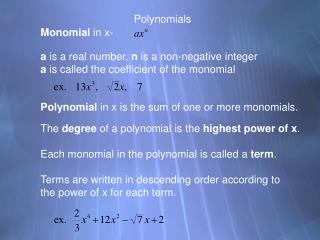DownloadDownload PresentationPolynomials

# Polynomials

Télécharger la présentation## Polynomials

- - - - - - - - - - - - - - - - - - - - - - - - - - - E N D - - - - - - - - - - - - - - - - - - - - - - - - - - -
##### Presentation Transcript

1. Polynomials Monomial in x- a is a real number, n is a non-negative integer a is called the coefficient of the monomial Polynomial in x is the sum of one or more monomials. The degree of a polynomial is the highest power of x. Each monomial in the polynomial is called a term. Terms are written in descending order according to the power of x for each term.

2. Degree of Polynomial Graph 0 1 2 3 Horizontal line Line with slope a Parabola facing up or down Curved, one end up other end down

3. Pre calculus Problem of the Day Homework p. p. 297 1-31 odds The effectiveness of a television commercial depends on How many times a viewer watches it. After some experiments, an advertising agency found that if the effectiveness, E, is measured on a scale of 1 to 10, then where n is the number of times a viewer watches a given commercial. For a commercial to have maximum effectiveness, how many times should a viewer watch it?

4. Polynomial Functions Degree 2 and higher. Will have end behavior as follows: Even degree: Odd degree: If leading coefficient is pos, If leading coefficient is pos, Both ends up Right side up, left side down If leading coefficient is neg, If leading coefficient is neg, Both ends down Right side down, left side up

5. Zeros and Multiplicity for Polynomial Functions Zeros of the function are the x intercepts or the x values that make the function equal zero. Each polynomial function will have a number of zeros equal to the degree of the polynomial. Zeros can be found by setting the function equal to zero and factoring when possible. Some factors are repeated. In this case the zero is said to have a multiplicity equal to the number of times the factor occurs. Zeros with even multiplicities are tangent points on the x-axis for the graph of the polynomial.

6. Find the following for the above polynomial: Describe the end behavior of the graph of f(x). f(0) f(-2) Zeros of the function. (factor)

7. Polynomial Functions Degree 2 and higher. For the following, n is the degree of the polynomial. Will have at most n zeros Will turn up to n-1 times Will have tangency points wherever a zero occurs an even number of times Will be symmetric with the y-axis if f(-x) = f(x). Will be symmetric with the origin if f(-x) = -f(x).

8. Intermediate Value Theorem For any polynomial f(x), and any interval [a,b]; If f(a) is positive and f(b) is negative, then there is a zero of the function between a and b. If f(a) is negative and f(b) is positive, then there is a zero of the function between a and b. Practice #18 p. 298 33 – 39 odds, 41 – 61 eoo